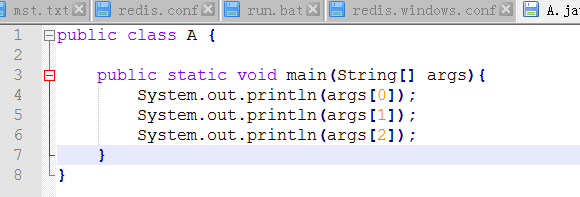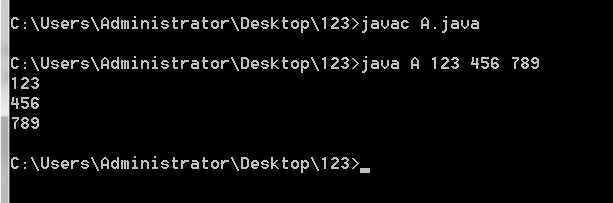java的main函数中的String args[]要怎么理解

5个回答

``````比如一个类 A.java

javac A.java
java A  "123" "456"   //你知道 运行的时候,这个地方是可以后面带参数的 参数接收了就可以在args获取
``````args是java保留的与控制相关的字符串数组的引用名，

eclipse运行的时候你会发现没这句话就没法run As java applicationsJava中构造函数File（String）未定义
package nmd; import java.io.*; public class FileHello { public static void main(String[] args) { try { Runtime ec=Runtime.getRuntime(); File file=new File("file.txt"); ec.exec(file.getAbsolutePath()); } catch (Exception e) { System.out.println(e); } } } 照着课本例题打的，以前忘了用的哪个版本的eclipse，是可以运行的，可现在换了版本是KEPLER的eclipse却无法运行，总是报错Exception in thread "main" java.lang.Error: Unresolved compilation problems: The constructor File(String) is undefined The method getAbsolutePath() is undefined for the type File at nmd.FileHello.main(FileHello.java:8) 求大神指点！
[java]关于main函数的执行先后顺序

java中静态代码块为什么不可以放在main函数里面！！！

# 在主函数中怎么写才能输出User中的值， 自己写的永远都是输出com.exam.bean.User@27f8302d。看不见值？ ```public User getUser(String grade) throws ClassNotFoundException, SQLException{ con=MysqlHelper.conn(); User user=new User(); String sql="select * from user where grade=?"; stmt=(PreparedStatement) con.prepareStatement(sql); stmt.setString(1, grade); rs=stmt.executeQuery(); if(rs.next()){ user.setId(rs.getInt(1)); user.setUsername(rs.getString(2)); user.setName(rs.getString(3)); user.setCode(rs.getString(4)); user.setGrade(rs.getString(5)); } rs.close(); stmt.close(); con.close(); return user; } public static void main(String[] args) throws SQLException, ClassNotFoundException, IOException { System.out.println(); }
java中的主函数后的定义语句

package complex_number; import java.util.*; public class ComplexNumber { public static void main(String[] args){ java.util.Scanner scanner = new java.util.Scanner(System.in); float m_dRealPart; float m_dImaginPart; System.out.println("Please enter the real and imaginary parts of the plural："); m_dRealPart=scanner.nextFloat(); m_dImaginPart=scanner.nextFloat(); System.out.println("\n"); System.out.println("What kind of operation do you want to do? (1 Plus, 2 minus, 3 multiply, 4 divide)"); } /*构造函数，ComplexNumber,将实部和虚部置0*/ public ComplexNumber(){ m_dRealPart=0; m_dImaginPart=0; } 请问在构造函数的时候为什么会错？
java中为什么只进行一个初始化就会执行类中的函数
import java.awt.*;import javax.swing.*; import java.awt.event.*; public class E2 extends Frame{ class WindowCloser extends WindowAdapter { public void windowClosing(WindowEvent we) {System.exit(0); } } E2() { super("简单图形"); setSize(200,200); setVisible(true); addWindowListener(new WindowCloser()); } public void paint(Graphics e) { e.drawLine(50,50,120,120); e.drawRect(50, 50, 70, 70); e.drawOval(70,70,90,90); } public static void main(String args[]) { new E2(); } } 代码如上，主main函数中知识一个初始化声明语句，并没有声明要执行 E2类中的paint函数，为什么程序运行时会直接画出图形啊。本人刚开始看java，中间都是大概看的，不太懂这个，谢谢指点。
java怎么动态化用户输入的函数原型求定积分
``` import java.util.Scanner; public class Demo{ /* * 用户输入区间 * 均分区间 * 获取到函数原型 * 每个区间的中点代入函数原型得到y * 每个区间的高度y的和乘以区间的长度 * 得到积分 * */ static float minX; static float maxX; static String function; static float num = 0;//区间高度之和 static float leng = 0;//区间的长度 static float centerNum = 0;//区间的中间值 public static void DingJifen(){ System.out.println("请输入要定积分的区间:\nmin(x) = "); minX = new Scanner(System.in).nextFloat(); System.out.println("max(x) = "); maxX = new Scanner(System.in).nextFloat(); System.out.println("请输入函数原型：\ny="); function = new Scanner(System.in).next(); leng = (maxX - minX)/1000; for(float a = minX;a<=maxX;a += leng){ centerNum = a+leng/2; num += function(centerNum); } System.out.println("在"+minX+"到"+maxX+"区间内，这个函数的定积分是："+(num*leng)); } // 获取函数原型 private static float function(float centerNum) { /* * 这个类不知道要怎么写 * 开始想的是将用户输入的函数原型 * 转化成ascill码然后循环判断类型最后整合起来 * 但是函数原型并不是确定的，可能会包含math函数或者其他的 * 好难啊。。。。 * */ float y; for(int i = 0;i<function.length();i++){ int a = function.charAt(i);//转换成ascll码 Object b = null; if (48<=a&&a<=57) { //a是数字 b = a; break; }else if(65<=a&&a<=90){ //a是大写字母 b = (char)a; break; }else if(97<=a&&a<=122){ //a是小写字母 b = (char)a; break; }else if(a==94){ //a是^ b = '^'; break; } if(a=='x'||a=='X'){ b = centerNum; } } return 0; } public static void main(String[] args) { DingJifen(); } } ```
JAVA中函数的参数值的传递问题

java怎么动态化用户输入的函数求定积分
``` import java.util.Scanner; public class Demo{ /* * 用户输入区间 * 均分区间 * 获取到函数原型 * 每个区间的中点带入函数原型得到高度y *高度y的和乘以均分的区间x * 得到积分 * */ static float minX; static float maxX; static String function; static float num = 0;//区间高度之和 static float leng = 0;//区间的长度 static float centerNum = 0;//区间的中间值 public static void DingJifen(){ System.out.println("请输入要定积分的区间:\nmin(x) = "); minX = new Scanner(System.in).nextFloat(); System.out.println("max(x) = "); maxX = new Scanner(System.in).nextFloat(); System.out.println("请输入函数原型：\ny="); function = new Scanner(System.in).next(); leng = (maxX - minX)/100; for(float a = minX;a<=maxX;a += leng){ centerNum = a+leng/2; num += function(centerNum); } System.out.println("在"+minX+"到"+maxX+"区间内，这个函数的定积分是："+(num*leng)); } // 获取函数原型 private static float function(float centerNum) { /* * 这个方法不知道怎么写了 * 想着把用户输入的函数原型转化成ascill码 * 然后循环遍历判断数据类型 * 最后整合起来 * 但是用户输入的函数里面可能有log、sqrt、sin等等 * 不知道怎么办了，是不是我思路有问题，求解！ * */ float y; for(int i = 0;i<function.length();i++){ int a = function.charAt(i);//转换成ascll码 Object b = null; if (48<=a&&a<=57) { //a是数字 b = a; break; }else if(65<=a&&a<=90){ //a是大写字母 b = (char)a; break; }else if(97<=a&&a<=122){ //a是小写字母 b = (char)a; break; }else if(a==94){ //a是^ b = '^'; break; } if(a=='x'||a=='X'){ b = centerNum; } } return 0; } public static void main(String[] args) { DingJifen(); } } ```

Java入口函数中调用静态方法传入参数的问题？
public class Main { public static void main(String [] args) { Main main = new Main(); String str = "abc" ; char[] array = {'a','b','c'}; change(str,array); System.out.println(str + " " + String.valueOf(array)); } public static void change(String str , char[] array) { str = "cba"; array = 'g'; } } ``` 运行结构为：abc gbc，不知道这是为啥？ ```
java中关于Sqrt（）函数
import java.math.*; public class Test { public static void main(String[] args) { BigDecimal b1 = new BigDecimal(Math.sqrt(5)-1,new MathContext(100)); BigDecimal b2 = new BigDecimal(2,new MathContext(100)); BigDecimal result = b1.divide(b2, new MathContext(100)); String str = result.toString(); int a=str.length(); System.out.println(a); System.out.print(str); } } 上面由于采用的是sqrt()函数而导致返回精度只有50位，现在想知道求一个数的开方后面的所有精度，比如根号2后面的1000位，该怎么求？
Java的死循环里的代码不执行

java中Date类型如何与mysql中的datetime类型联系

Java学习的正确打开方式

linux系列之常用运维命令整理笔录

Vue + Spring Boot 项目实战（十四）：用户认证方案与完善的访问拦截

Python十大装B语法
Python 是一种代表简单思想的语言，其语法相对简单，很容易上手。不过，如果就此小视 Python 语法的精妙和深邃，那就大错特错了。本文精心筛选了最能展现 Python 语法之精妙的十个知识点，并附上详细的实例代码。如能在实战中融会贯通、灵活使用，必将使代码更为精炼、高效，同时也会极大提升代码B格，使之看上去更老练，读起来更优雅。 1. for - else 什么？不是 if 和 else 才

2019年11月中国大陆编程语言排行榜
2019年11月2日，我统计了某招聘网站，获得有效程序员招聘数据9万条。针对招聘信息，提取编程语言关键字，并统计如下： 编程语言比例 rank pl_ percentage 1 java 33.62% 2 c/c++ 16.42% 3 c_sharp 12.82% 4 javascript 12.31% 5 python 7.93% 6 go 7.25% 7

SQL-小白最佳入门sql查询一

“狗屁不通文章生成器”登顶GitHub热榜，分分钟写出万字形式主义大作

IT界知名的程序员曾说：对于那些月薪三万以下，自称IT工程师的码农们，其实我们从来没有把他们归为我们IT工程师的队伍。他们虽然总是以IT工程师自居，但只是他们一厢情愿罢了。 此话一出，不知激起了多少(码农)程序员的愤怒，却又无可奈何，于是码农问程序员。 码农：你知道get和post请求到底有什么区别？ 程序员：你看这篇就知道了。 码农：你月薪三万了？ 程序员：嗯。 码农：你是怎么做到的? 程序员：
《程序人生》系列-这个程序员只用了20行代码就拿了冠军

11月8日，由中国信息通信研究院、中国通信标准化协会、中国互联网协会、可信区块链推进计划联合主办，科技行者协办的2019可信区块链峰会将在北京悠唐皇冠假日酒店开幕。 　　区块链技术被认为是继蒸汽机、电力、互联网之后，下一代颠覆性的核心技术。如果说蒸汽机释放了人类的生产力，电力解决了人类基本的生活需求，互联网彻底改变了信息传递的方式，区块链作为构造信任的技术有重要的价值。 　　1

Android 9.0系统新特性，对刘海屏设备进行适配

【技巧总结】位运算装逼指南

8年经验面试官详解 Java 面试秘诀
作者 | 胡书敏 责编 | 刘静 出品 | CSDN（ID：CSDNnews） 本人目前在一家知名外企担任架构师，而且最近八年来，在多家外企和互联网公司担任Java技术面试官，前后累计面试了有两三百位候选人。在本文里，就将结合本人的面试经验，针对Java初学者、Java初级开发和Java开发，给出若干准备简历和准备面试的建议。   Java程序员准备和投递简历的实

1.两种思维方式在求职面试中，经常会考察这种问题：北京有多少量特斯拉汽车？ 某胡同口的煎饼摊一年能卖出多少个煎饼？ 深圳有多少个产品经理？ 一辆公交车里能装下多少个乒乓球？ 一